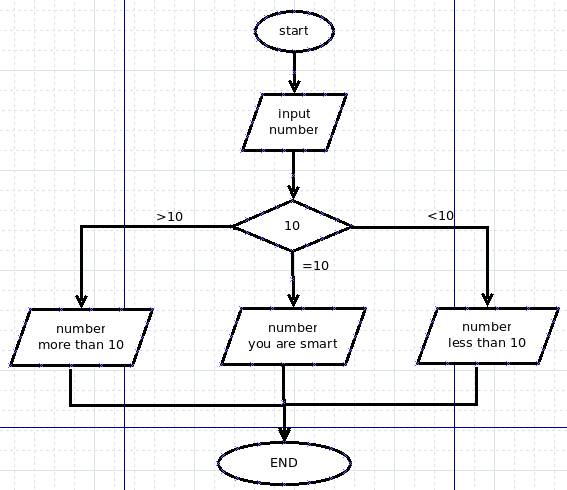# 语句(2)

## if

if，其含义就是：conj. （表条件）如果。if 发起的就是一个条件，它是构成条件语句的关键词。

``````>>> a = 8
>>> if a==8:
...     print a
...
8``````

`if a==8:`，这句话里面如果条件 `a==8` 返回的是 True，那么就执行下面的语句。特别注意，冒号是必须的。下面一行语句 `print a` 要有四个空格的缩进。这是 Python 的特点，称之为语句块。

Python 开发者有意让远反了缩排規則的程序不能通过编译，以此来强迫程序员养成良好的编程习惯。並且Python 語言利用缩排表示语句块的开始和结束（Off-side 规则），而非使用花括号或者某种关键字。增加缩表示语句块的开始，而減少缩排则表示语句块的结束。缩排成为了语法的一部分。例如 if 语句.

• 必须要通过缩进方式来表示语句块的开始和结束
• 缩进用四个空格（也是必须的，别的方式或许可以，但不提倡）

## if/else/elif``````if 条件 1:
执行的内容 1
elif 条件 2:
执行的内容 2
elif 条件 3：
执行的内容 3
else:
执行的内容 4``````

elif 用于多个条件时使用，可以没有。另外，也可以只有 if，而没有 else。

``````#! /usr/bin/env python
#coding:utf-8

print "请输入任意一个整数数字："

number = int(raw_input())   #通过 raw_input()输入的数字是字符串
#用 int()将该字符串转化为整数

if number == 10:
print "您输入的数字是：%d"%number
print "You are SMART."
elif number > 10:
print "您输入的数字是：%d"%number
print "This number is more than 10."
elif number < 10:
print "您输入的数字是：%d"%number
print "This number is less than 10."
else:
print "Are you a human?"    ``````

``````\$ Python num.py

12

This number is more than 10.

\$ Python num.py

10

You are SMART.

\$ Python num.py

9

This number is less than 10.``````

``#! /usr/bin/env python``

## 三元操作符

``````>>> name = "qiwsir" if "laoqi" else "github"
>>> name
'qiwsir'
>>> name = 'qiwsir' if "" else "python"
>>> name
'Python'
>>> name = "qiwsir" if "github" else ""
>>> name
'qiwsir'``````

• 如果 X 为真，那么就执行 A=Y
• 如果 X 为假，就执行 A=Z

``````>>> x = 2
>>> y = 8
>>> a = "python" if x>y else "qiwsir"
>>> a
'qiwsir'
>>> b = "python" if x<y else "qiwsir"
>>> b
'python'``````